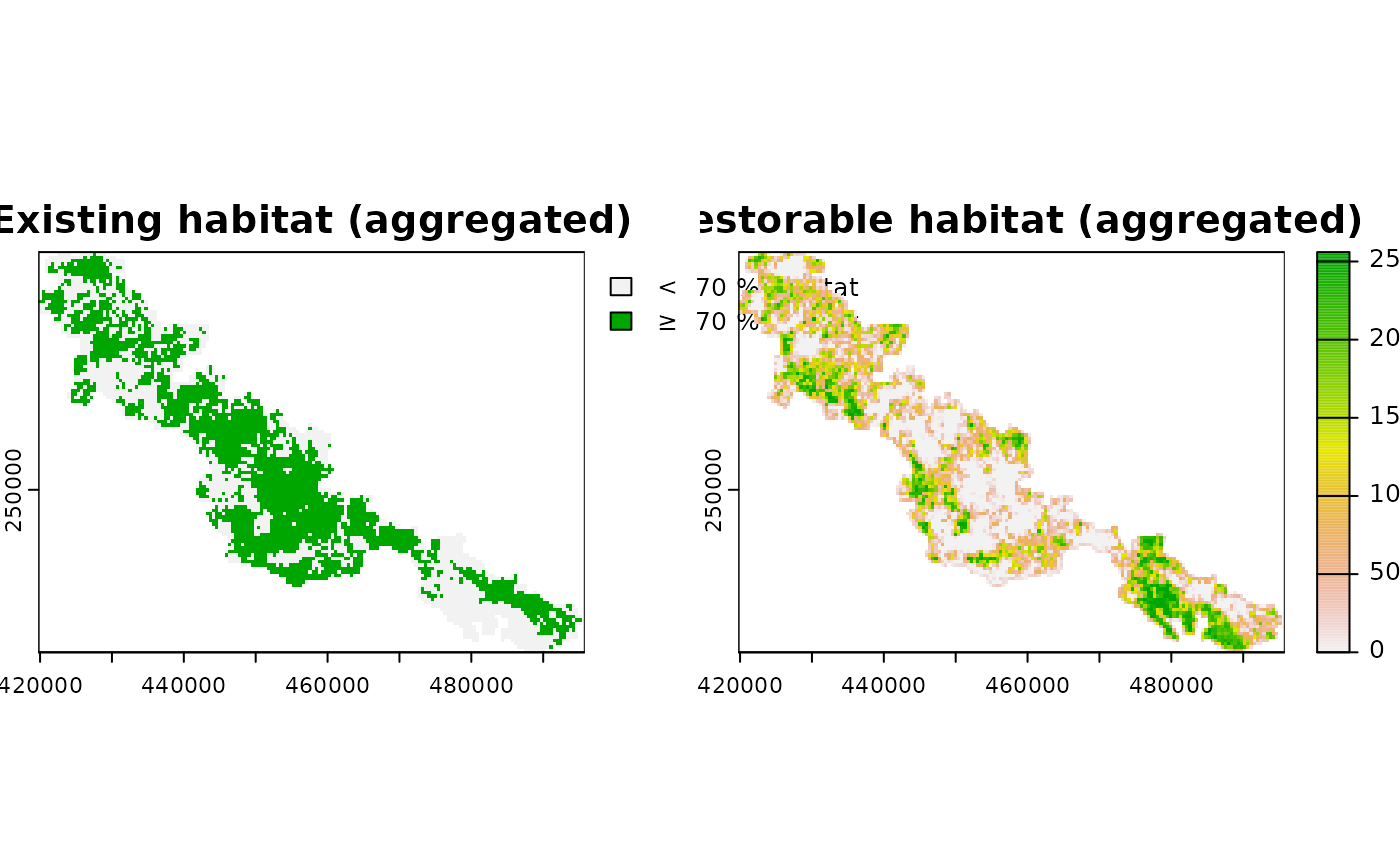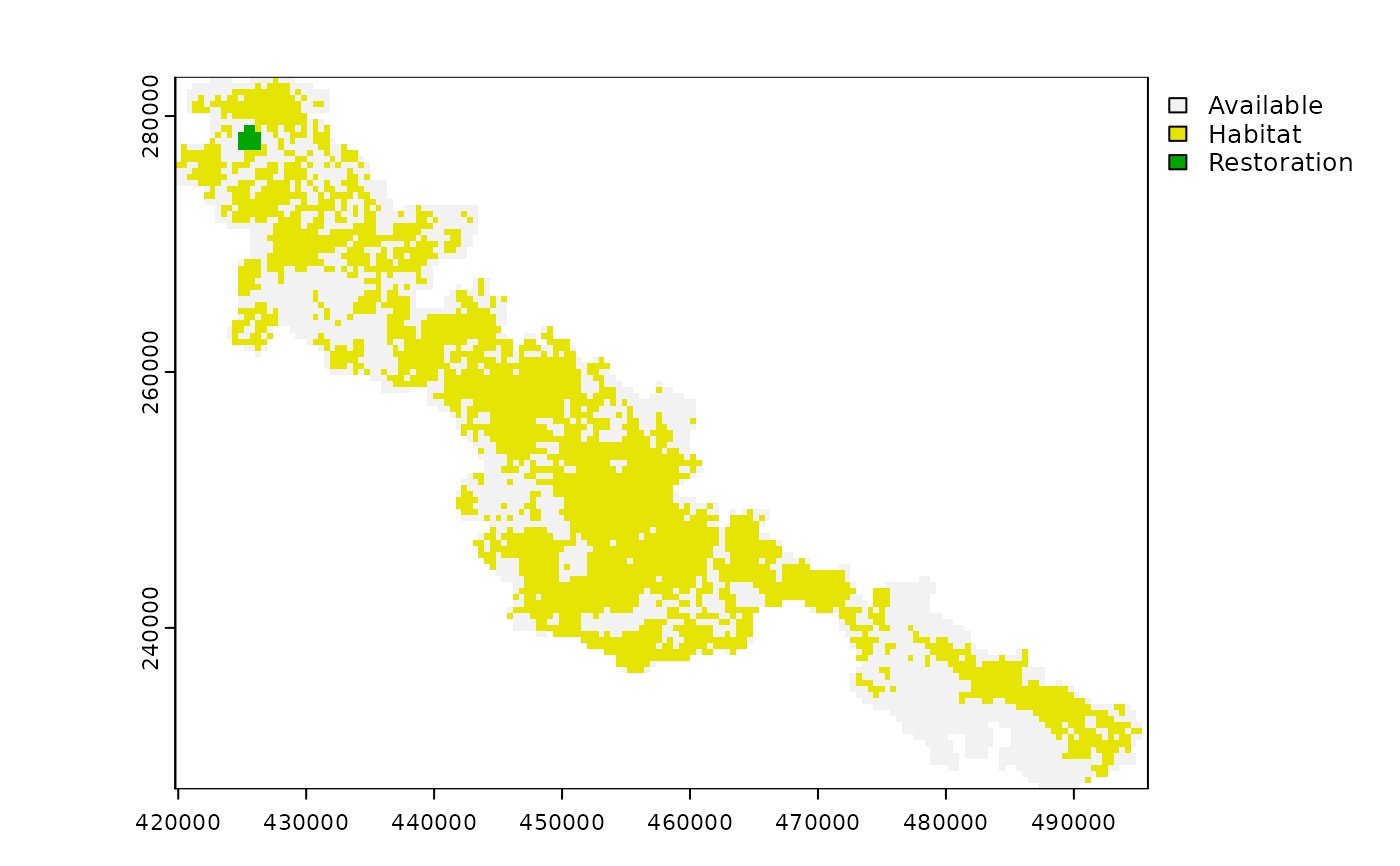Add constraint to a restoration problem (restopt_problem()) object to specify the compactness of a solution.

## Usage

add_compactness_constraint(problem, max_diameter, unit = "m")

## Arguments

problem

restopt_problem() Restoration problem object.

max_diameter

numeric Maximum diameter value.

unit

unit object or a character that can be coerced to an area unit (see unit package), or "cells" for cell width of aggregated habitat raster. Corresponds to the unit of the maximum diameter. If the input habitat raster does not use a projected coordinate system, only "cells" is available.

## Value

An updated restoration problem (restopt_problem()) object.

## Details

The compactness constraint is defined according to the diameter of the smallest enclosing circle which contains the center of selected planning units for restoration (see https://w.wiki/4vfg). The unit of the diameter corresponds either to a unit available in the unit package, or to planning unit width ("cells"). Note that, as the computation occurs on aggregated cells, if max_diameter is used with a different unit than "cells", it will be rounded to the closest corresponding number of cells. For example, a diameter of 4 cells means that no more than 4 cells can be found in line in the solution. In practice, this constraint is useful to ensure the feasibility of a restoration project, and to integrate economies of scale. Compact restoration areas are usually associated with lower costs and easier management, because it ensures that restoration sites are not too far away from each other (e.g. lower travel costs between sites, less areas to monitor, etc.).

Other constraints: add_available_areas_constraint(), add_components_constraint(), add_connected_constraint(), add_locked_out_constraint(), add_min_iic_constraint(), add_min_mesh_constraint(), add_restorable_constraint()

## Examples

# \donttest{
habitat_data <- rast(
system.file("extdata", "habitat_hi_res.tif", package = "restoptr")
)

# create problem
p <- restopt_problem(
existing_habitat = habitat_data,
aggregation_factor = 16,
habitat_threshold = 0.7
) %>%
min_restore = 200,
max_restore = 300,
) %>%

# plot preprocessed data
plot(rast(list(p$data$existing_habitat, p$data$restorable_habitat)), nc = 2)# print problem
print(p)
#> -----------------------------------------------------------------
#>                          Restopt
#> -----------------------------------------------------------------
#> original habitat:     habitat_hi_res.tif
#> aggregation factor:   16
#> habitat threshold:    0.7
#> existing habitat:     in memory
#> restorable habitat:   in memory
#> -----------------------------------------------------------------
#> objective:            No optimization objective
#> -----------------------------------------------------------------
#> constraints:
#>   -  restorable (min_restore = 200, max_restore = 300, min_proportion = 1, unit = ha)
#>   -  compactness (max_diameter = 1800, unit = m)
#> -----------------------------------------------------------------
#> settings:
#>   - precision = 4
#>   - time_limit = 0
#>   - nb_solutions = 1
#>   - optimality_gap = 0
#>   - solution_name_prefix = Solution
#> -----------------------------------------------------------------

# Solve problem
s <- solve(p)
#> Good news: the solver found 1 solution satisfying the constraints ! (solving time = 0.06 s)
# plot solution
plot(s)# }